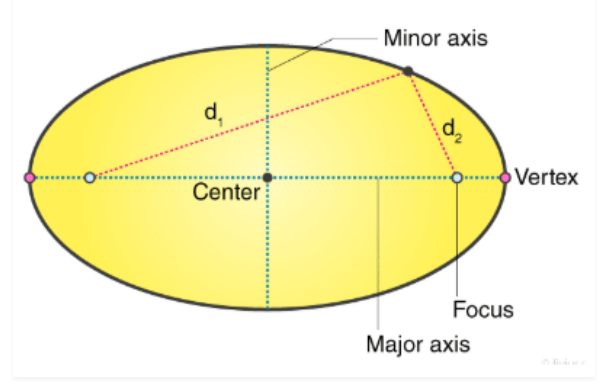# Properties of Ellipse

The set of all points on a plane whose distance from two points add up to a constant is known as an Ellipse. It is one of the conic sections which is obtained by the intersection of a right circular cone. The fixed point on the Ellipse is the focus and the fixed straight line is the directrix. The constant ratio of the Ellipse is called the eccentricity. As far as the JEE exam is concerned, Ellipse is one of the important topics. In this article, we will learn the important properties of the Ellipse.

 The standard equation of an Ellipse is given by $\frac{x^2}{ a^2} +\frac{y^2}{ b^2} = 1$## 10 Important Properties of the Ellipse

The following are the important properties of the Ellipse.

1. Center: The point of intersection of the major axis and the minor axis is the center.

2. Focus: The fixed point on the Ellipse is called the focus.

3. Major Axis: The longest diameter of the Ellipse.

4. Minor Axis: The shortest diameter of the Ellipse.

5. Directrix: The fixed straight line of the Ellipse is the directrix.

6. Eccentricity: The constant ratio of the Ellipse denoted by e. e<1.

7. Tangent: The line which touches the Ellipse at just one point is called the tangent.

8. Chord: The line joining any two points on the Ellipse is the chord.

9. Latus rectum: The chord which passes through the focus and perpendicular to the major axis.

10. As per the Lahire’s Theorem: Let P and P’ be fixed lines in the plane, and let us consider a another moving line on which any 3 points A, A’ and A” are pointed. If we constrain A and A’ to lie in P and P’ respectively, then A” describes an ellipse.

### Example

Determine the equation of the Ellipse whose directrices along y = ± 9 and foci at (0, ± 4). Also, find the length of its latus rectum.

Solution:

The equation of the Ellipse is given by $\frac{x^2}{ a^2} +\frac{y^2}{ b^2} = 1$.

Given coordinates of the foci are (0, ± 4).

Coordinates of foci are (0, ± be).

Equation of directrix y = ± be

Therefore, be = 4 …(i)

b/e = 9 …(ii)

Multiply (i) and (ii), we get

b2 = 36

b = 6

a2 = b2(1-e2)

= b2-(be)2

= 36 – 42

= 20

The required equation of the Ellipse is (x2/20 ) + (y2/36) = 1.

Length of latus rectum = 2a2/b = 2(20/6) = 20/3.